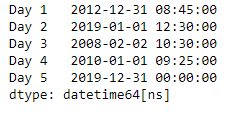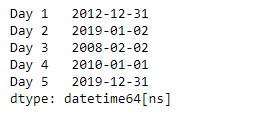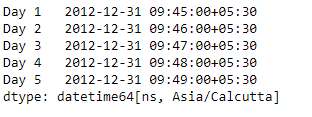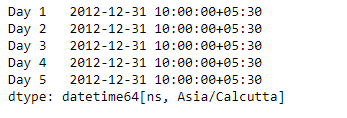# Python | Pandas Series.dt.round

• Last Updated : 20 Mar, 2019

`Series.dt` can be used to access the values of the series as datetimelike and return several properties. Pandas` Series.dt.round()` function perform round operation on the data to the specified freq.

Syntax: Series.dt.round(*args, **kwargs)

Parameter :
freq : The frequency level to round the index to

Returns : DatetimeIndex, TimedeltaIndex, or Series

Example #1: Use `Series.dt.round()` function to round the datetime data of the given series object to the specified frequency.

 `# importing pandas as pd``import` `pandas as pd`` ` `# Creating the Series``sr ``=` `pd.Series([``'2012-12-31 08:45'``, ``'2019-1-1 12:30'``, ``'2008-02-2 10:30'``,``               ``'2010-1-1 09:25'``, ``'2019-12-31 00:00'``])`` ` `# Creating the index``idx ``=` `[``'Day 1'``, ``'Day 2'``, ``'Day 3'``, ``'Day 4'``, ``'Day 5'``]`` ` `# set the index``sr.index ``=` `idx`` ` `# Convert the underlying data to datetime ``sr ``=` `pd.to_datetime(sr)`` ` `# Print the series``print``(sr)`

Output :Now we will use `Series.dt.round()` function to round the datetime values in the given series object to Daily frequency.

 `# round to daily frequency``result ``=` `sr.dt.``round``(freq ``=` `'D'``)`` ` `# print the result``print``(result)`

Output :As we can see in the output, the `Series.dt.round()` function has successfully rounded the datetime values in the given series object to the specified frequency.

Example #2 : Use `Series.dt.round()` function to round the datetime data of the given series object to the specified frequency.

 `# importing pandas as pd``import` `pandas as pd`` ` `# Creating the Series``sr ``=` `pd.Series(pd.date_range(``'2012-12-31 09:45'``, periods ``=` `5``, freq ``=` `'T'``,``                            ``tz ``=` `'Asia / Calcutta'``))`` ` `# Creating the index``idx ``=` `[``'Day 1'``, ``'Day 2'``, ``'Day 3'``, ``'Day 4'``, ``'Day 5'``]`` ` `# set the index``sr.index ``=` `idx`` ` `# Print the series``print``(sr)`

Output :Now we will use `Series.dt.round()` function to round the datetime values in the given series object to Hourly frequency.

 `# round to hourly frequency``result ``=` `sr.dt.``round``(freq ``=` `'H'``)`` ` `# print the result``print``(result)`

Output :As we can see in the output, the `Series.dt.round()` function has successfully rounded the datetime values in the given series object to the specified frequency.

My Personal Notes arrow_drop_up